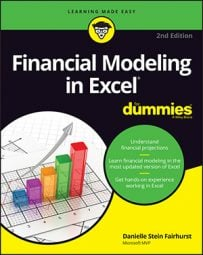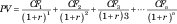##### Financial Modeling in Excel For DummiesKnowing how the discounted cash flow (DCF) valuation works is good to know in financial modeling. The core concept of the DCF is that of the basic finance concept of the time value of money, which states that money is worth more in the present than the same amount in the future. In other words, a dollar today is worth more than a dollar tomorrow.

The idea behind the time value of money is that if you have to wait to receive your funds, you’re missing out on other potential investment opportunities, not to mention the risk of not receiving the money at all. If you have to wait to get your money, you’d expect some compensation — hence, the concept of interest.

For example, if you invest \$100 at a 10 percent annual interest rate today, it will be worth \$110 in one year. Conversely, \$110 in one year would only be worth \$100 today. In this example, the 10 percent is referred to as the discount rate. As the name suggests, the discount rate is a key input you need to calculate the DCF.

A DCF valuation uses a modeler’s projections of future cash flow for a business, project, or asset and discounts this cash flow by the discount rate to find what it’s worth today. This amount is called the present value (PV). Excel has a built-in function that automatically calculates PV.

If you want to know the math behind the function, here’s how to calculate PV, where CF is the cash flow for the projected year, r is the discount rate, and n is the number of years in the future:In the case of a project or asset with finite cash flows, the modeler would forecast all the cash flows and discount them to find the present value. For example, if you were purchasing an asset such as a large piece of machinery that has an expected life of ten years, you would model the entire ten years. When purchasing a business, however, which is expected to be a going concern and the cash flows are expected to continue into perpetuity, a DCF analysis must find a terminal value at the end of a certain forecast period instead of forecasting cash flows into eternity.

The terminal value represents the projected value of the company or asset at the end of the forecast period. Forecast periods are typically projected to the point at which cash flows are expected to grow at a stable and predictable rate. When the cash flows become stable, you can then reasonably estimate a fair value for the steady cash flows that are earned after that point in time.

There are variations of the DCF analysis in which the cash flows, discount rates, and terminal values can differ, but the most common method is to project free cash flow to firm, find a terminal value using the perpetuity growth method, and discount these values by the business’s weighted average cost of capital.

Download the file File 1101.xlsx, open it, and spend a few minutes reviewing and familiarizing yourself with the financial statements.

To perform a DCF analysis, you need several years of financial statements, which are provided in this new model.